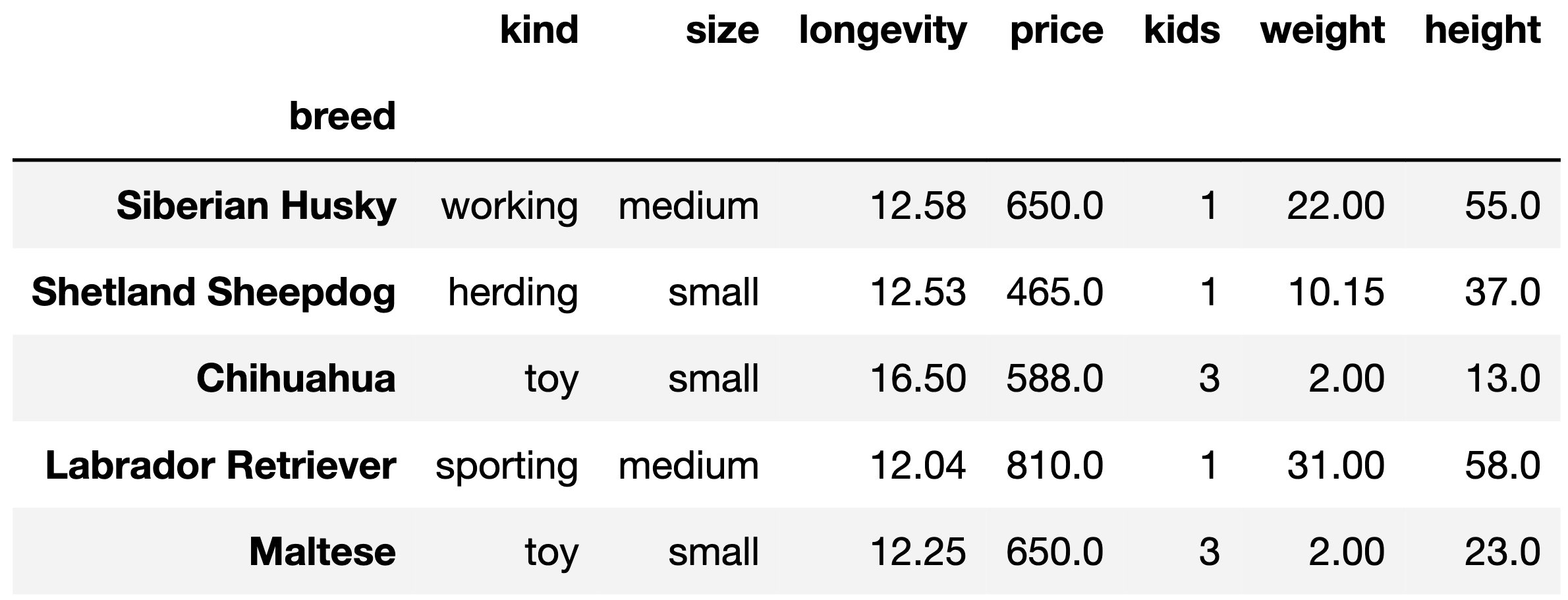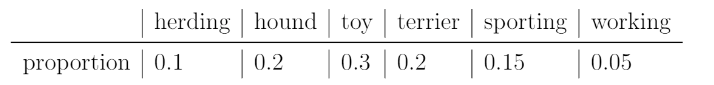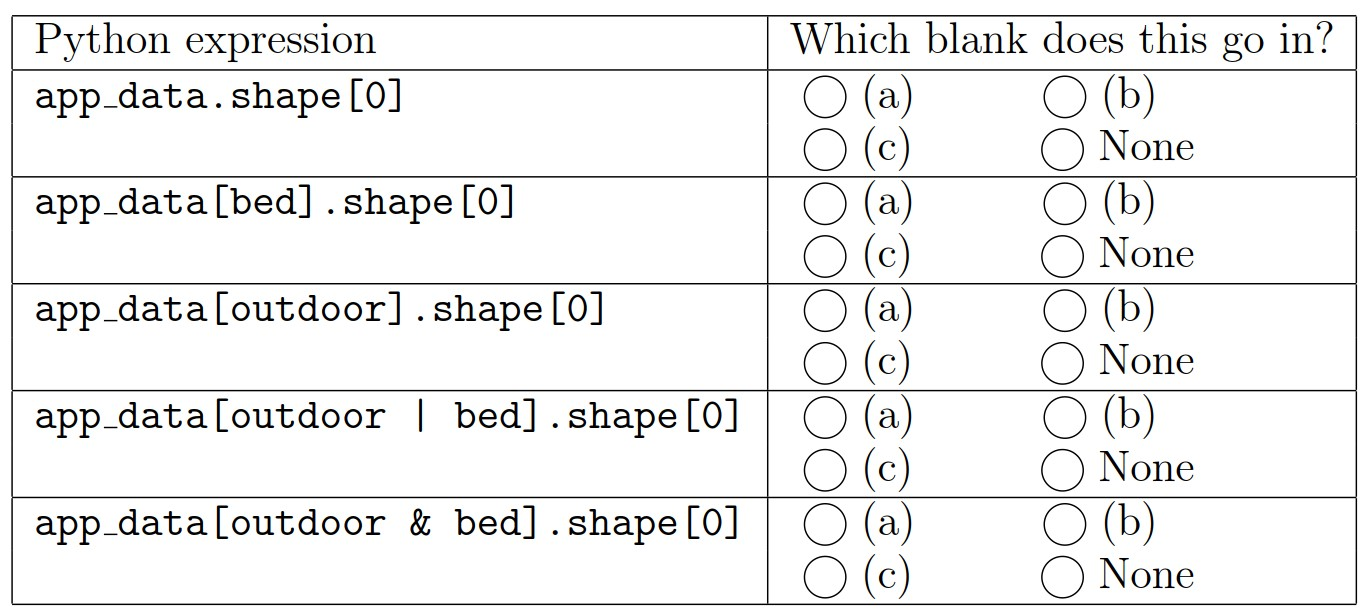# Discussion 6: Midterm Solutions and Hypothesis Testing

The problems in this worksheet are taken from past exams. Work on them on paper, since the exams you take in this course will also be on paper.

We encourage you to complete this worksheet in a live discussion section. Solutions will be made available after all discussion sections have concluded. You don’t need to submit your answers anywhere.

Note: We do not plan to cover all problems here in the live discussion section; the problems we don’t cover can be used for extra practice.

## Problem 1

For Problem 1 we will be using this DataFrame:

The American Kennel Club (AKC) organizes information about dog breeds. We’ve loaded their dataset into a DataFrame called df. The index of df contains the dog breed names as str values.

The columns are:

• 'kind' (str): the kind of dog (herding, hound, toy, etc.). There are six total kinds.
• 'size' (str): small, medium, or large.
• 'longevity' (float): typical lifetime (years).
• 'price' (float): average purchase price (dollars).
• 'kids' (int): suitability for children. A value of 1 means high suitability, 2 means medium, and 3 means low.
• 'weight' (float): typical weight (kg).
• 'height' (float): typical height (cm).

The rows of df are arranged in no particular order. The first five rows of df are shown below (though df has many more rows than pictured here).Assume: - We have already run import babypandas as bpd and import numpy as np.

Every year, the American Kennel Club holds a Photo Contest for dogs. Eric wants to know whether toy dogs win disproportionately more often than other kinds of dogs. He has collected a sample of 500 dogs that have won the Photo Contest. In his sample, 200 dogs were toy dogs.

Eric also knows the distribution of dog kinds in the population:### Problem 1.1

Select all correct statements of the null hypothesis.

• The distribution of dogs in the sample is the same as the distribution in the population. Any difference is due to chance.

• Every dog in the sample was drawn uniformly at random without replacement from the population.

• The number of toy dogs that win is the same as the number of toy dogs in the population.

• The proportion of toy dogs that win is the same as the proportion of toy dogs in the population.

• The proportion of toy dogs that win is 0.3.

• The proportion of toy dogs that win is 0.5.

### Problem 1.2

Select the correct statement of the alternative hypothesis.

• The model in the null hypothesis underestimates how often toy dogs win.

• The model in the null hypothesis overestimates how often toy dogs win.

• The distribution of dog kinds in the sample is not the same as the population.

• The data were not drawn at random from the population.

### Problem 1.3

Select all the test statistics that Eric can use to conduct his hypothesis.

• The proportion of toy dogs in his sample.

• The number of toy dogs in his sample.

• The absolute difference of the sample proportion of toy dogs and 0.3.

• The absolute difference of the sample proportion of toy dogs and 0.5.

• The TVD between his sample and the population

### Problem 1.4

Eric decides on this test statistic: the proportion of toy dogs minus the proportion of non-toy dogs. What is the observed value of the test statistic?

• -0.4

• -0.2

• 0

• 0.2

• 0.4

### Problem 1.5

Which snippets of code correctly compute Eric’s test statistic on one simulated sample under the null hypothesis? Select all that apply. The result must be stored in the variable stat. Below are the 5 snippets

Snippet 1:

a = np.random.choice([0.3, 0.7])
b = np.random.choice([0.3, 0.7])
stat = a - b

Snippet 2:

a = np.random.choice([0.1, 0.2, 0.3, 0.2, 0.15, 0.05])
stat = a - (1 - a)

Snippet 3:

a = np.random.multinomial(500, [0.1, 0.2, 0.3, 0.2, 0.15, 0.05]) / 500
stat = a - (1 - a)

Snippet 4:

a = np.random.multinomial(500, [0.3, 0.7]) / 500
stat = a - (1 - a)

Snippet 5:

a = df.sample(500, replace=True)
b = a[a.get("kind") == "toy"].shape / 500
stat = b - (1 - b)
• Snippet 1

• Snippet 2

• Snippet 3

• Snippet 4

• Snippet 5

### Problem 1.6

After simulating, Eric has an array called sim that stores his simulated test statistics, and a variable called obs that stores his observed test statistic.

What should go in the blank to compute the p-value?

np.mean(sim _______ obs)
• <

• <=

• ==

• >=

• >

### Problem 1.7

Eric’s p-value is 0.03. If his p-value cutoff is 0.01, what does he conclude?

• He rejects the null in favor of the alternative.

• He accepts the null.

• He accepts the aleternative.

• He fails to reject the null.

## Problem 2

For this question, let’s think of the data in app_data as a random sample of all IKEA purchases and use it to test the following hypotheses.

Null Hypothesis: IKEA sells an equal amount of beds (category 'bed') and outdoor furniture (category 'outdoor').

Alternative Hypothesis: IKEA sells more beds than outdoor furniture.

The DataFrame app_data contains 5000 rows, which form our sample. Of these 5000 products,

• 1000 are beds,
• 1500 are outdoor furniture, and
• 2500 are in another category.

### Problem 2.1

Which of the following could be used as the test statistic for this hypothesis test? Select all that apply.

• Among 2500 beds and outdoor furniture items, the absolute difference between the proportion of beds and the proportion of outdoor furniture.

• Among 2500 beds and outdoor furniture items, the proportion of beds.

• Among 2500 beds and outdoor furniture items, the number of beds.

• Among 2500 beds and outdoor furniture items, the number of beds plus the number of outdoor furniture items.

### Problem 2.2

Let’s do a hypothesis test with the following test statistic: among 2500 beds and outdoor furniture items, the proportion of outdoor furniture minus the proportion of beds.

Complete the code below to calculate the observed value of the test statistic and save the result as obs_diff.

    outdoor = (app_data.get('category')=='outdoor')
bed = (app_data.get('category')=='bed')
obs_diff = ( ___(a)___ - ___(b)___ ) / ___(c)___

The table below contains several Python expressions. Choose the correct expression to fill in each of the three blanks. Three expressions will be used, and two will be unused.### Problem 2.3

Suppose we generate 10,000 simulated values of the test statistic according to the null model and store them in an array called simulated_diffs. Complete the code below to calculate the p-value for the hypothesis test.

    np.count_nonzero(simulated_diffs _________ obs_diff)/10000

What goes in the blank?

• <

• <=

• >

• >=